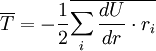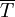Virial theorem$\overline{T}= - \frac{1}{2} \overline{\sum_i \frac{dU}{dr}\cdot r_i}$
where$\overline{T}$ is the kinetic energy. The overlines represent time averages. The right hand side is known as the virial of Clausius (Ref. 2).